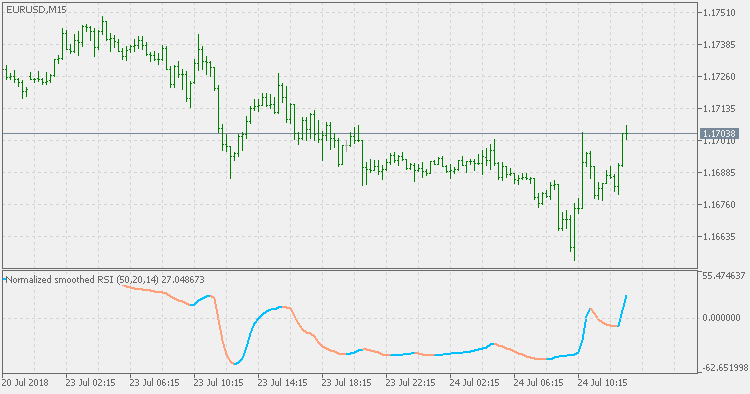Interesting script?
So post a link to it -
let others appraise it

You liked the script? Try it in the MetaTrader 5 terminal# Normalized RSI JMA Smoothed - indicator for MetaTrader 5

Views:
1263
Rating:
Published:
2018.07.25 12:55

Normalized RSI tends to be "nervous" (the direction of the RSI itself, and of the normalized RSI as a result is changing sometimes erratically).

This version is adding JMA smoothing to it in order to make that volatility lesser and to attempt to make the slope of the RSI more usable without adding significant lag.PS: if the normalization period is set to <=1, then no normalization of the RSI is performed, and in that case you shall have a "simple" RSI.Normalized RSI

Normalized RSI tries to fix the "RSI problem": the longer the calculating period, the flatter the RSI becomes.Price Zone Oscillator - Floating Levels

Compared to the Price Zone Oscillator indicator, this version is using floating levels to find out the significant levels.Sigmoidal Normalized RSI

This is sigmoidal normalized version of RSI. Additional JMA smoothing is used in order to produce smooth results.Price Zone Oscillator - Smoothed

This version of Price Zone Oscillator is an attempt to solve the "too fast" slope issue of the original indicator.